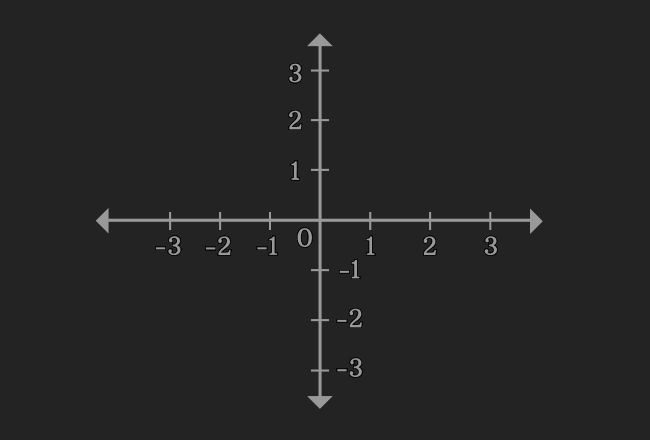# System of Linear equations

A collection of two or more linear equations that have same set of variables is called a system of linear equations.

## Introduction

The meaning of system is a set of connected things. In some cases, two or more linear equations have a connection and the relationship between them can be understood from their same set of variables.

#### Example

$x+3y-5 = 0$ and $7x-8y-6 = 0$ are two linear equations.The two linear equations have a connection and it is they both have same set of variables $x$ and $y$. Therefore, a collection of such linear equations is called as system of linear equations and it is simply called as linear system.

When $x = 2$ and $y = 1$, the two linear equations are satisfied simultaneously.

1. $2+3(1)-5 = 0$
2. $7(2)-8(1)-6 = 0$

Therefore, the system of linear equations $x+3y-5 = 0$ and $7x-8y-6 = 0$ are called as simultaneous linear equations.

### Methods of solving

The system of simultaneous linear equations can be solved in three different methods.

Latest Math Topics
Jun 26, 2023
Jun 23, 2023

###### Math Questions

The math problems with solutions to learn how to solve a problem.

Learn solutions

Practice now

###### Math Videos

The math videos tutorials with visual graphics to learn every concept.

Watch now

###### Subscribe us

Get the latest math updates from the Math Doubts by subscribing us.